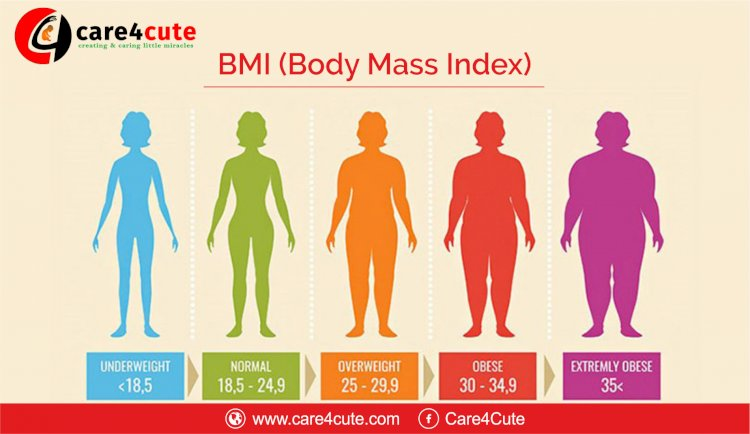# What is Body Mass Index (BMI) and How to Calculate BMI?

## Body Mass Index is a simple calculation using a person's height and weight. Learn about What is Body Mass Index (BMI) and How to Calculate BMI.Body Mass Index, or BMI, is a person’s weight in kilograms divided by the square of his/her height in meters. The National Institute of Health (NIH) has now defined BMI to be the deciding parameter to know if you are underweight, normal weight, overweight or obese.

What Is BMI?

BMI (body mass index) is a calculated number representing a person's level of fat or obesity level. According to the Centers for Disease Control and Prevention (CDC), a BMI of 30 or above indicates obesity. BMI levels are broken down by weight range and are as follows:

BMI < 18.5 is underweight
BMI 18.5-24.9 is normal weight
BMI 25-29.9 is overweight
BMI 30-39.9 indicates obesity
BMI > 40 indicates morbid obesity

In children, age and gender are considered in tables that are produced for growth charts by the CDC. However, BMI is calculated with the same formula for both children and adults. In general, children are assessed as being underweight if they fall below the 5th percentile and overweight/obese if they fall above the 95th percentile.

## Calculating BMI: The Formula

The formula to calculate BMI requires information about a person's height in meters and weight in kilograms. For weight in pounds and height in inches, there is a different formula, but due to rounding, it may not produce the exact same results. The BMI formulas are:

BMI = weight (in kilograms) / height (in meters) ^2

or

BMI = weight (in pounds) / height (in inches) ^2 * 703

1) How to use
BMI calculator?

- Enter your weight and select its unit (kilograms or pounds).
- Enter your height in feet and inches.
- Press calculate.
- If you want to calculate again, simply press re-calculate.

2) How is BMI calculated?

BMI is calculated by dividing a person’s weight in kilograms by the square of his/her height in meters. For example, if your weight is 60 kilograms and your height is 5 feet 3 inches, your BMI will be calculated as follows:

60 / 2.61 (1.6 * 1.6)
(5 feet 3 inches is approximately 1.6 meters)

This gives us a BMI reading at 22.9.

## Example BMI Calculations

If you have your weight in pounds, as most of us in the U.S. do, you'll need to convert the weight to kilograms first. The formula to convert pounds to kilograms is dividing the weight in pounds by 2.2. To convert inches to meters, you take the height in inches and multiply it by 0.0254.

Let's do a calculation. A man weighs 220 pounds and is 70 inches tall. We will perform the BMI calculation using both ways to show how to use the formulas.

#### English Units

BMI = weight (in pounds) / height (in inches) ^2 * 703

• (220 / 70^2) * 703
• (220 / 4900) * 703
• 0.045 * 703
• BMI = 31.63

#### Metric Units

BMI = weight (in kilograms) / height (in meters) ^2

• 220 lbs / 2.2 = 100 kg
• 70 in * 0.0254 = 1.78 m
• 100 / 1.78^2
• 100 / 3.17
• BMI = 31.55